# Earthquake data and Benford’s Law

Much has been written about Benford's Law, that weird phenonmenon where if you have a naturally occuring set of numerical data, 30% of the numbers will begin with 1, 18% will begin with 2, 12% will begin with 3, and so on. You might expect the distribution of leading digits to be uniformly distributed, but no, that just isn't the case. 60% of the time the leading digit is 1, 2, or 3. Though it may seem like some curious mathematical anomaly, Benford's Law has been used to detect fraud in elections and accounting.

In this post let's use R to verify that earthquake data obtained from the USGS does indeed follow Benford's Law. On May 28, 2016, I downloaded data for all earthquakes in the past 30 days. The data has a number of fields, including earthquake magnitude, depth of the earthquake, location, time and many others. Benford's Law says those numbers should follow a particular distribution.

The formula for Benford's Law is as follows:

$P(d) = \log_{10} \left(1+\frac{1}{d}\right)$

That says the probability that a digit d occurs as the first number is equal the log base 10 of 1 + 1/d. We can quickly generate these for d = 1 – 9 in R:

log10(1 + (1/(1:9)))

##  0.30103000 0.17609126 0.12493874 0.09691001 0.07918125 0.06694679
##  0.05799195 0.05115252 0.04575749


And we can make a quick plot as follows:

barplot(log10(1 + (1/(1:9))), names.arg = 1:9, main = "Benford's Law")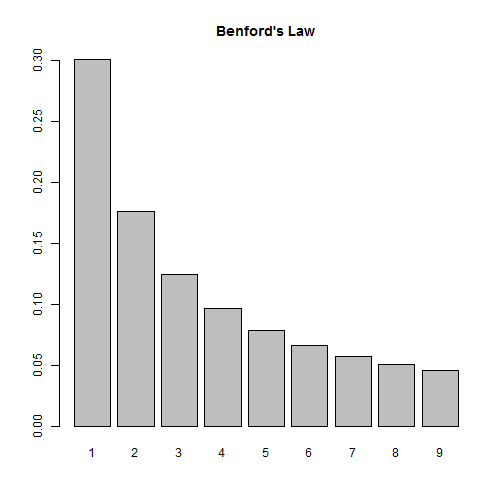So according to this law, if we look at the distribution of first digits in our earthquake data, we should see them closely follow this distribution. Let's find out!

First we need to import the data. Thanks to the USGS, this data comes ready for analysis. All we need to do is read it into R:

dat <- read.csv("all_month.csv")
nrow(dat)

##  8584


Over 8500 earthquakes in the past 30 days! A quick look at the magnitude shows us most of them are very small:

summary(dat$mag)  ## Min. 1st Qu. Median Mean 3rd Qu. Max. NA's ## -1.900 0.790 1.280 1.526 1.930 7.200 20  This also tells us we have some negative numbers (?) as well as some missing data, NA's. We also have numbers that start with 0 and have a decimal, such as 0.79. (In this case, Benford would say the number “starts” with a 7, not a 0.) This means when we determine the leading digits, we'll need to ignore negative signs, leading 0s, decimals and missing values. Let's investigate at the mag column. First we remove the NA's. Below is.na(dat$mag) generates a logical vector of TRUE and FALSE values. Adding an ! in front reverses the TRUE and FALSE values. Finally inserting the result in subsetting brackets returns only those values that are TRUE, ie not missing.

digits <- dat$mag[!is.na(dat$mag)]


Next we extract the first digits. To help with this I use two R packages, magrittr and stringr. The magrittr package allows us to “chain” commands together with the %>% operator. The stringr package provides the str_extract function that allows us to extract phrases that follow a certain pattern. So what we have below can be translated as follows:

• take the absoluet value of digits (to get rid of negative signs)
• convert digits to character (so we can use the next two functions)
• extract anything that is not a “0” or a “.” (We express this a regular expression: "[^0\\.]")
• extract the first digit (starting and ending at position 1)
library(magrittr)
library(stringr)
digits <- abs(digits) %>%
as.character() %>%
str_extract(pattern = "[^0\\.]") %>%
substr(1,1)


As an extra precaution, we then convert digits to a factor and set levels to be all digits 1 through 9. This ensure all digits are represented in subsequent calculations.

digits <- factor(digits, levels = 1:9) # ensure all digits represented


And finally we tally the first digits and calculate proportions:

table(digits) %>% prop.table()

## digits
##          1          2          3          4          5          6
## 0.42800141 0.17134139 0.05738763 0.09447248 0.05809177 0.03990142
##          7          8          9
## 0.04459570 0.05304542 0.05316277


We see 1 appearing a lot more often, but it's hard to tell how the other digits compare to Benford's Law. Let's put both distributions on the same graph. To do this, I went ahead and created a function that will allow us to check any vector of numbers against Benford's Law. Let's load the function and see how it works, then we'll break it down and explain it.

library(ggplot2)
compareBenford <- function(d){
digits <- d[!is.na(d)]
digits <- substr(stringr::str_extract(as.character(abs(digits)), pattern = "[^0\\.]"),1,1)
digits <- factor(digits, levels = 1:9) # ensure all digits represented
depth <- prop.table(table(digits))
ben <- log10(1 + (1/(1:9)))
dat2 <- data.frame(ben, depth)
names(dat2) <- c("Benford","Digit",deparse(substitute(d)))
dat2L <- reshape2::melt(dat2,id.vars="Digit", variable.name = "Type", value.name = "Frequency")
ggplot(dat2L, aes(x=Digit, y=Frequency, fill=Type)) +
geom_bar(stat = "identity", position = "dodge")
}

compareBenford(dat$mag)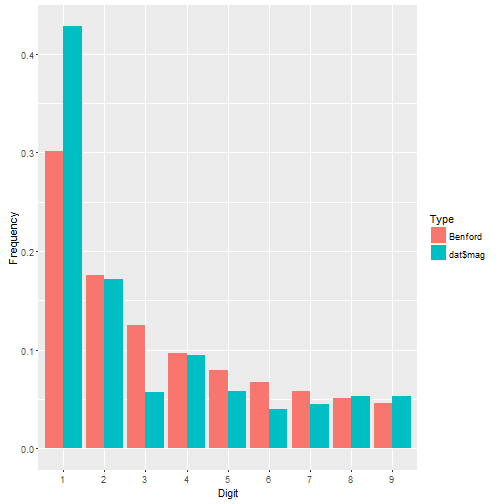We see dat$mag has more 1's than we might expect and fewer 3's, but otherwise seems to follow the distribution pretty closely. Let's check out earthquake depth.

compareBenford(dat$depth)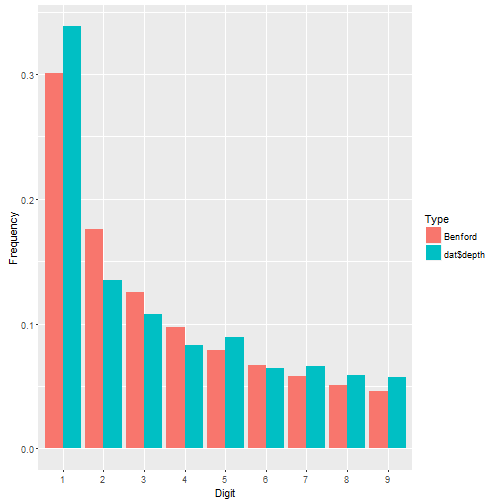This appears to fit even better. About the function: • the first four lines are what we did before. Notice I went ahead and nested the functions instead of using magrittr. That's how I originally coded it before deciding to write a blog post. Then I decided to break out magrittr for the fun of it. • After that I calculate Benford's proportions. • Then I put both sets of proportions in a data frame. • Then I change the names of the data frame. Notice there are three names to change, not two. Why? The depth vector is actually a table. When it gets pulled into a data frame, two columns are produced: the table cells and the table names. The names are the digits 1 – 9. Notice I also use deparse(substitute(d)) to name the first-digit proportions in the data frame. This ensures that whatever vector I give my function, the name of it will be the vector itself. So if I give it dat$mag, the column name of the first-digit proportions will be dat\$mag.
• Next I reshape the data using the melt function in the reshape2 package. This puts all the proportions in one column called Frequency and the type of proportion in another column called Type. I still have my Digit column that indicates which proportion goes with which digit. Having my data in this form allows me to use ggplot to map fill color to Type.
• Finally I create the graph. I set Digit on the x-axis, Frequency on the y-axis, and map Type to the fill color. The geom_bar function says draw a bar plot. The stat = "identity" and position = "dodge" arguments say set the height of the bars to the actual values and “dodge” them so they're next to one another.

Let's look at ALL numeric columns in the earthquakes data. We need to identify all numeric columns and pull them into a vector for our function to work. Here's how I do it:

theCols <- sapply(dat, class) %in% c("numeric","integer")
numCols <- names(dat)[theCols]
allDigits <- unlist(dat[,numCols])

compareBenford(allDigits)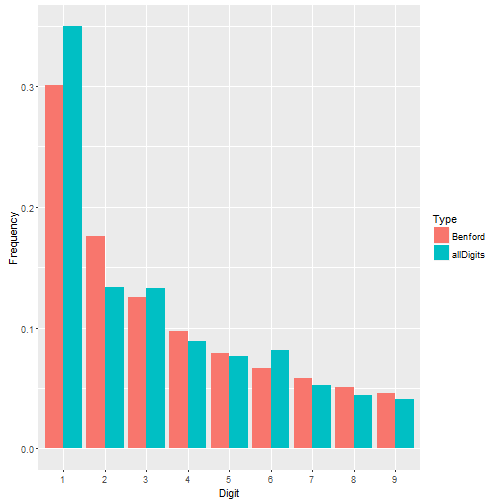Not a bad fit. Benford's Law seems to work just fine for the earthquake data.

But what about data where Benford's Law doesn't work? Recall that Benford's Law applies to most naturally occuring sets of numbers. Not all. Take the iris data that come with R. These are measurements of 50 flowers from each of 3 species of iris. This is a small data set looking at very specific measurements. It doesn't seem to follow Benford's Law very well.

irisDigits <- unlist(iris[,1:4])
compareBenford(irisDigits)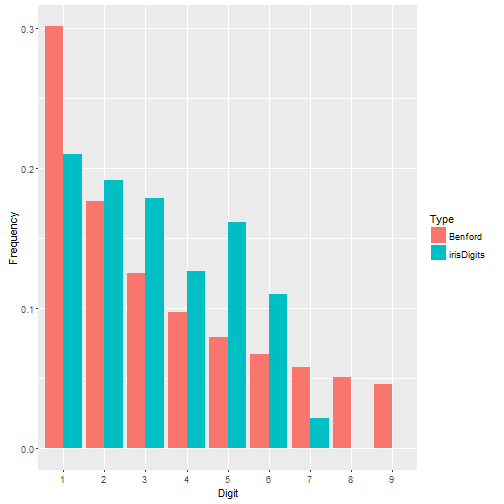This site uses Akismet to reduce spam. Learn how your comment data is processed.